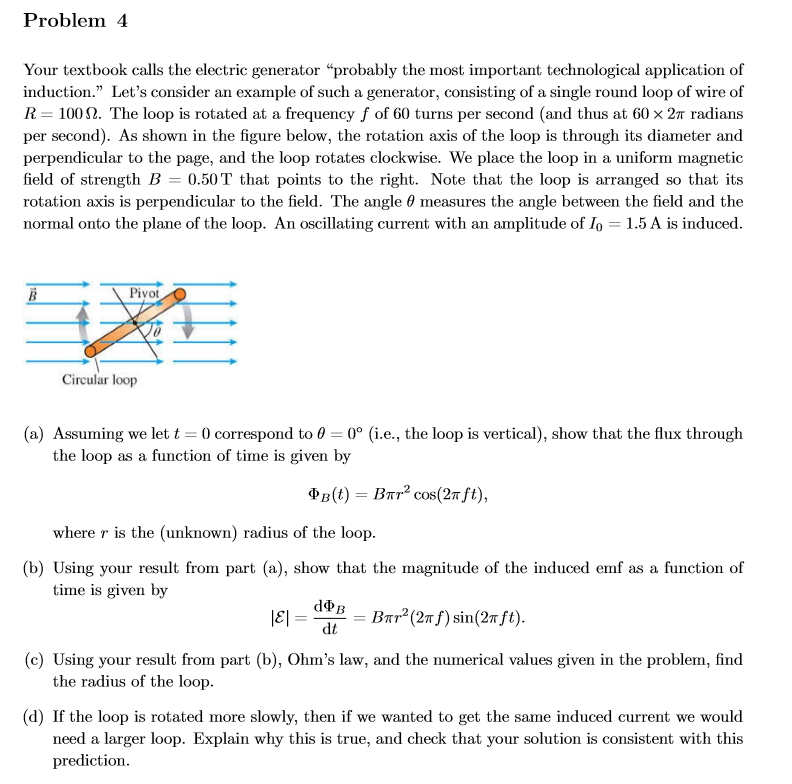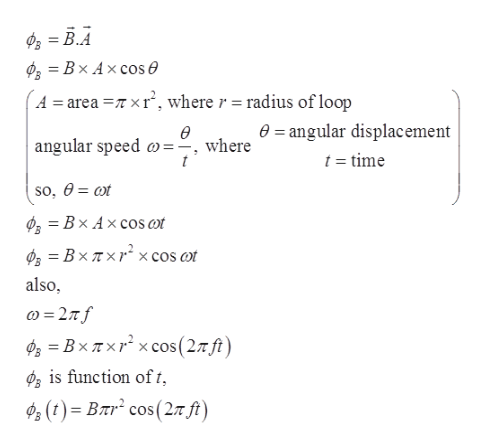# Problem 4Your textbook calls the electric generator "probably the most important technological application ofinduction." Let's consider an example of such a generator, consisting of a single round loop of wire ofR 100. The loop is rotated at a frequency f of 60 turns per second (and thus at 60 x 2T radiansper second). As shown in the figure below, the rotation axis of the loop is through its diameter andperpendicular to the page, and the loop rotates clockwise. We place the loop in a uniform magneticfield of strength B 0.50T that points to the right. Note that the loop is arranged so that itsrotation axis is perpendicular to the field. The angle 0 measures the angle between the field and thenormal onto the plane of the loop. An oscillating current with an amplitude of Io = 1.5 A is inducedPiyotВCircular loop(a) Assuming we let t 0 correspond to 0 0° (i.e., the loop is vertical), show that the flux throughthe loop as a function of time is given byФв (0) — Впr? сos(2т ft),where r is the (unknown) radius of the loop(b) Using your result from part (a), show that the magnitude of the induced emf as a function oftime is given bydФв|E =Bar2(27fsin(2Tft)dt(c) Using your result from part (b), Ohm's law, and the numerical values given in the problem, findthe radius of the loop(d) If the loop is rotated more slowly, then if we wanted to get the same induced current we wouldneed a larger loop. Explain why this is true, and check that your solution is consistent with thisprediction

Question
39 viewshelp_outlineImage TranscriptioncloseProblem 4 Your textbook calls the electric generator "probably the most important technological application of induction." Let's consider an example of such a generator, consisting of a single round loop of wire of R 100. The loop is rotated at a frequency f of 60 turns per second (and thus at 60 x 2T radians per second). As shown in the figure below, the rotation axis of the loop is through its diameter and perpendicular to the page, and the loop rotates clockwise. We place the loop in a uniform magnetic field of strength B 0.50T that points to the right. Note that the loop is arranged so that its rotation axis is perpendicular to the field. The angle 0 measures the angle between the field and the normal onto the plane of the loop. An oscillating current with an amplitude of Io = 1.5 A is induced Piyot В Circular loop (a) Assuming we let t 0 correspond to 0 0° (i.e., the loop is vertical), show that the flux through the loop as a function of time is given by Фв (0) — Впr? сos(2т ft), where r is the (unknown) radius of the loop (b) Using your result from part (a), show that the magnitude of the induced emf as a function of time is given by dФв |E = Bar2(27fsin(2Tft) dt (c) Using your result from part (b), Ohm's law, and the numerical values given in the problem, find the radius of the loop (d) If the loop is rotated more slowly, then if we wanted to get the same induced current we would need a larger loop. Explain why this is true, and check that your solution is consistent with this prediction fullscreen
check_circle

Step 1

Since we only answer up to 3 sub-parts, we’ll answer the first 3. Please resubmit the question and specify the other subparts (up to 3) you’d like answered.

Step 2

Given:

Resistance of wire loop R = 100 Ω

Rotational frequency of the loop is f = 60 turns/s = 60×2π rad/s

Magnitude of magnetic field B = 0.50 T

Angle between rotation axis and magnetic field = θ

Induced oscillating current Io = 1.5 A

Step 3

(a)

The flux through the l...help_outlineImage TranscriptioncloseBx Ax cos e A = area T xr, where r =radius of loop 0= angular displacement ө angular speed w=-, where t t =time so, @ %3D оx Bx Ax cos a x cos ot BxTx also BxTxr xcos(27ft) is function of t, ф. (t) - Влr* сos(2л ft) fullscreen

### Want to see the full answer?

See Solution

#### Want to see this answer and more?

Solutions are written by subject experts who are available 24/7. Questions are typically answered within 1 hour.*

See Solution
*Response times may vary by subject and question.
Tagged in

### Physics